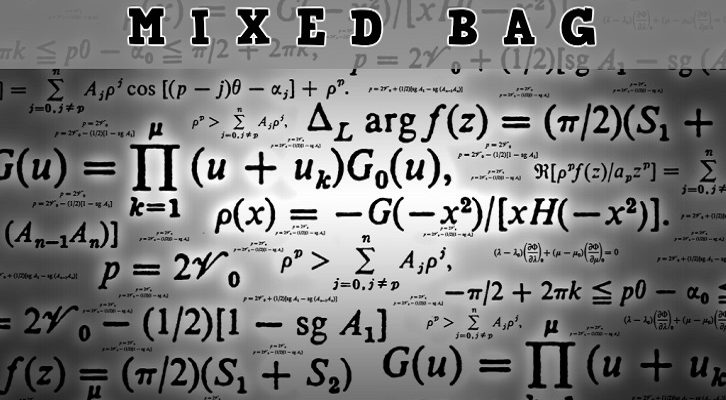# Few more questions – assorted topics

Have given below a few more questions from assorted topics

1. A number n! is written in base 6 and base 8 notation. Its base 6 representation ends with 10 zeroes. Its base 8 representation ends with 7 zeroes. Find the smallest n that satisfies these conditions. Also find the number of values of n that will satisfy these conditions.

2. [x] is the greatest integer less than or equal to x. Find the number of positive integers n such that [n/11] = [n/13].

3. Positive numbers 1 to 55, inclusive are placed in 5 groups of 11 numbers each. What is the maximum possible average of the medians of the 5 groups?

## One comment

1.deepak says:

Answer of 3 – (50+44+38+32+26)/5=38